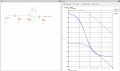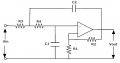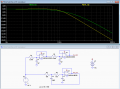# 2nd Order passive Low Pass Filter

Thread Starter

#### Alexandru Chiser

Joined Feb 16, 2017
44
Hello,

I need to design a 2nd order RC low pass filter for a PWM that has a frequency of 488.28Hz. The DC component is at the lowest frequency, so my cut-off frequency of the filter should be as small as possible. Until now i designed a 2nd order filter like this: R1=R2=10K and C1=C2=470nF. Calculating the cut-off frequency it results 33Hz (good enough) - i have simulated this circuit but the cut-off is at 14Hz and i also implemented in real life and i still get 14Hz. It has to do with the parasitic resistance/capacitance of the components. But this is not why i am here.

The output of this 2nd order filter is connected at the input of an OP-AMP (so its a HIGH impedance input). I have seen on some website that i should increase R2 10 times, and decrease C2 10 times, it has something to do with the impedance and cascading 2 1st order filters. I know how to calculate the input/output impedance of a 1st order filter, but i dont know how they influence each other. How important are these impedances, and how can i achieve the best results using this filter?!

What should i consider in building it?

PS: when R1=R2 and C1=C2 the Q factor was 0.33 (using an online calculator), but increasing R2 10 times, and decreasing C2 10 times, the Q factor was 0.54...
PS2: i would prefer to keep these values (10K and/or 100K and 470nF and/or 47nF) - i already own these components and i dont need/want to order new ones.

Thanks in advance!

#### crutschow

Joined Mar 14, 2008
27,168
The problem with using two passive RC filters in cascade is that the impedance of the second affects the rolloff of the second (basically the first capacitor sees the parallel combination of the two resistors).
So if you make the second filter with ten times the impedance of the first, it will have less effect on the rolloff of the first and the total rolloff will be closer to what you calculated.

But if you have an op amp, why not make it into a 3-pole active filter for a much sharper and faster rolloff as shown here?
Note that 2-pole active filters can have a problem of feeding through spikes from the fast rise/fall times of the PWM signal. This 3-pole filter does not have that problem due to the passive RC low-pass input which immediately rolls off the sharp edges.

Last edited:
Thread Starter

#### Alexandru Chiser

Joined Feb 16, 2017
44
The board is already done and theres no time left to redraw the layout and send the gerbers. Id like to know how to calculate these impedances and demonstrate how they affect the filter.

Lets stick to this case: R1=10K and C1=470nF + R2=100K and C2=47nF. How do i demonstrate that this configuration is better than using same values for Rs and Cs? Also why did the Q factor got bigger?

#### crutschow

Joined Mar 14, 2008
27,168
Lets stick to this case: R1=10K and C1=470nF + R2=100K and C2=47nF. How do i demonstrate that this configuration is better than using same values for Rs and Cs? Also why did the Q factor got bigger?
You can demonstrate by calculating the transformer function of both or, since I'm lazy, I would show the difference on a simulator.
I use the free LTspice from Linear Technology/Analog Devices.

I assume the Q factor got larger because it has a sharper rolloff.

#### crutschow

Joined Mar 14, 2008
27,168
For the magnitude of the impedance (forgetting about phase shifts for the moment and concentrating on amplitude), you can redraw the circuit as all resistors.
But that won't give you the correct output voltage.
For example the 3-dB point (.7) gain of an RC filter is where the magnitude of the capacitance impedance equals the resistor resistance.
If you convert the capacitor impedance to a resistance without considering the phase-shift you would get a gain of 6dB not 3dB.

#### AnalogKid

Joined Aug 1, 2013
9,244
Turns out that sleep-deprivation and complex algebra do not mix. I deleted the post. Last week was wife's spine surgery #6. She's sore, I'm fried, stuff I learned in high school is out the window.

oops.

ak

•-live wire-
Thread Starter

#### Alexandru Chiser

Joined Feb 16, 2017
44
How can the transfer function help me with demonstrating the impact of the impedance?

I substitute Xc with 1/jwC and do the maths (voltage dividers) up to the output, and then?

PS: if you have any simulation, feel free to post it

Last edited:

#### crutschow

Joined Mar 14, 2008
27,168
How can the transformer function help me with demonstrating the impact of the impedance?
I was referring to the transfer function, that is, the output voltage response divided by the input voltage.
In this case that gives an equation with complex numbers (phasors).
But that's beyond my pay grade.Thread Starter

#### Alexandru Chiser

Joined Feb 16, 2017
44
The transfer function of the first one is:

Vx/Vin = 1/(sR1C1+1)

The second one:

Vout/Vx = 1/(sR2C2+1)

So:

Vin/Vout = 1/(sR1C1+1) * 1/(sR2C2+1) = 1/[(sR1C1+1)*(sR2C2+1)] = 1/(S^2R1C1R2C2 + SR1C1 + SR2C2 + 1)

But some webpages say that the transfer function is:

1/[S^2R1R2C1C2+S(R1C1+R1C2+R2C2)+1] OR 1/[S^2R1R2C1C2+S(R1C1+R2C1+R2C2)+1]

Where does this R1C2 or R2C1 term come from?

LE: i have found this http://www.johnhearfield.com/RC/RC4.htm but it still dont get it.

LE2: also this one: https://electronics.stackexchange.c...ency-response-of-2nd-order-rc-low-pass-filter this explains why theres a new term... So this is the transfer function of the filter

Last edited:
Thread Starter

#### Alexandru Chiser

Joined Feb 16, 2017
44
Using the LE2 i posted above i get this:

(1+sR2C2) / (s^2R1C1R2C2 + s(R1C1 + R1C2 + R2C2) +1) and i have this sR2C2 term...

It gets me confused...

LE: it seems that this transfer function is for a different filter. The correct one is the first one in the post above, the one that doesnt have the sR2C2 and R1C2 terms

Last edited:
Thread Starter

#### Alexandru Chiser

Joined Feb 16, 2017
44
Lets leave beside the transfer function, and concetrate on the impedane stuff. Why should i increase R2 10 times and decrease C2 10 times? How does this help me? How does the impedances (and how do i calculate them) influence the system.

The PWM comes from a microncontroller, and the output of the filter is connected to an op-amp (HIGH impedance)

#### crutschow

Joined Mar 14, 2008
27,168
Why should i increase R2 10 times and decrease C2 10 times?
The value of 10 is arbitrary.
The idea is to have the second filter impedance cause as little change as possible to the first filter response.
Ideally you could put an op amp follower between them for perfect isolation.
How does the impedances (and how do i calculate them) influence the system.
You put the values in your transfer function for both situations and calculate the difference in the output response.

Are you sure you don't just want to simulate the differences and just let the computer do all the calculations for you?Last edited:
Thread Starter

#### Alexandru Chiser

Joined Feb 16, 2017
44
I actually need the theory behind all of this. I need to demonstrate using formulas and calculus that this filter is good enough:

What is the transfer function?
What is the cut-off frequency and phase?
Let’s suppose i make r2=10*r1 and c1=10*c2, why is this better? How does that impedance stuff help me?

Thread Starter

#### Alexandru Chiser

Joined Feb 16, 2017
44
I will use a op-amp buffer between the stages, to separate them!

This is the final schematic of the filter system.

In the simulation you can see 4 curves: 2 of them are identical (the phase) and 2 are for the frequency response. The one that starts from arround 10dB is the one that doesnt use an OP-AMP, and the lower one is for the configuration in the photo. Why does it start from -120dB? It has to do with the OA, but how?

Also one more question: if i want to have a 3.3V maximum output from the filter, I need to apply a 3.3V to the OA supply pins, right?Apparently it doesnt matter if the supply voltage of the OP-AMP is 3.3V or 5V, so i will stick to 5V cus its the main supply voltage for the board.

How does this high input impedance of the OP-AMP and zero output impedande help isolate the filters between them?

Last edited:
Thread Starter

#### Alexandru Chiser

Joined Feb 16, 2017
44
I played a little bit with simulations and i discovered this:

Without OA: Fc = 12Hz and Phase = -52*
With OA: Fc = 22Hz and Phase = -66*

Also, using the OA i can achieve more voltage at the output. Without it i achieve a maximum of 3.28, and with it 3.286 (not much difference) (note that my uCs amplitude of the PWM is 3.287)

#### crutschow

Joined Mar 14, 2008
27,168
Why does it start from -120dB? It has to do with the OA, but how?
Also one more question: if i want to have a 3.3V maximum output from the filter, I need to apply a 3.3V to the OA supply pins, right?
You have only a single supply voltage so the op amp has no gain at 0V DC bias on the input.
You either need to use AC coupling and bias it a 1/2 the supply voltage, or use a dual supply.

3.3Vac is not 3.3V peak. For 3.3Vac rms from the filter, the signal is 3.3 x 1.4 x 2 = 9.2Vpp, so you need a single supply of 10V, with the input biased at 5V or dual ±5V supplies.

Thread Starter

#### Alexandru Chiser

Joined Feb 16, 2017
44
If i will implement the schematic from above, the one with the op-amp, why dont i use this one:But without R1 and R2. The inverting input will be connected directly to the output.

Is this configuration better? I mean.. if i change the project, at least lets make it better!

PS: Argh... dont mind this configuration. Ill stick to the one with the OA between the stages as an insolator!

PS2: ive added a 2nd supply, of -3.3V and the simulation of the amplitude starts from 10.34 db (the equivalent of 3.3V in db). In real life, i will not be able to add a -3.3V or -5V supply.

Will it make any difference in real life if i have 1 or 2 supply voltages? I see that the response is exactly the same, but the only difference is that one starts from 10.34dB and the other one from -120dB

Last edited:
Thread Starter

#### Alexandru Chiser

Joined Feb 16, 2017
44
If my PWM coming from the uC is between 0 and 3.3V i dont need +-5 supply voltage on the op-amp, i can use only +5V and GND. Right?

#### crutschow

Joined Mar 14, 2008
27,168
If my PWM coming from the uC is between 0 and 3.3V i dont need +-5 supply voltage on the op-amp, i can use only +5V and GND. Right?
Right.
But for proper AC simulation you need to add a small positive DC bias to the AC signal to bias the amp into its active region.
Is this considered a 2nd order active low pass filter? If not, what is it called... i know it`s active because it has an OA.
Just because it has an op amp doesn't mean it's an active filter.
It's two first-order passive filters in series with a buffer between the two filters.

To be considered an active filter, the opamp should have some positive feedback to shape the response. giving a 2nd order (or higher) filter with a sharper corner rolloff than two single-pole filters in series.

Here's an LTspice simulation comparison between two passive 1-pole in series with buffers and a Sallen-Key 2-pole butterworth active filter, both with the same -3dB frequency.
Note the sharper rolloff of the active filter.
Although they both eventually reach the same 12db/octave rolloff of a 2-pole filter, the active filter has about 9dB more attenuation at any given frequency after reaching the constant rolloff point.
(Also note the 1Vdc bias added to the AC source for the simulation).Last edited: